﻿

### On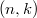$(n,k)$-quasi class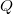$Q$ Operators

#### Abstract

Let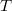$T$ be a bounded linear operator on a complex Hilbert space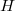$H$. In this paper we introduce a new class of operators:$(n,k)$-quasi class$Q$ operators, superclass of$(n,k)$-quasi paranormal operators. An operator$T$ is said to be$(n,k)$-quasi class$Q$ if it satisfies
for all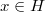$x\in H$ and for some nonnegative integers$n$ and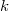$k$. We prove the basic structural properties of this class of operators. It will be proved that If$T$ has a no non-trivial invariant subspace, then the nonnegative operator
is a strongly stable contraction. In section 4, we give some examples which compare our class with other known classes of operators and as a consequence we prove that$(n,k)$-quasi class$Q$ does not have SVEP property. In the last section we also characterize the$(n,k)$-quasi class$Q$ composition operators on Fock spaces.

DOI Code: 10.1285/i15900932v39n2p39

Keywords: $(n,k)$-quasi class $Q$; $(n,k)$-quasi paranormal operators; SVEP property; Fock space; composition operators

Full Text: PDF